The leading digit of a number is the first digit that is not a zero.

Leading digit approximation only uses this digit and the other digits are replaced with a zero.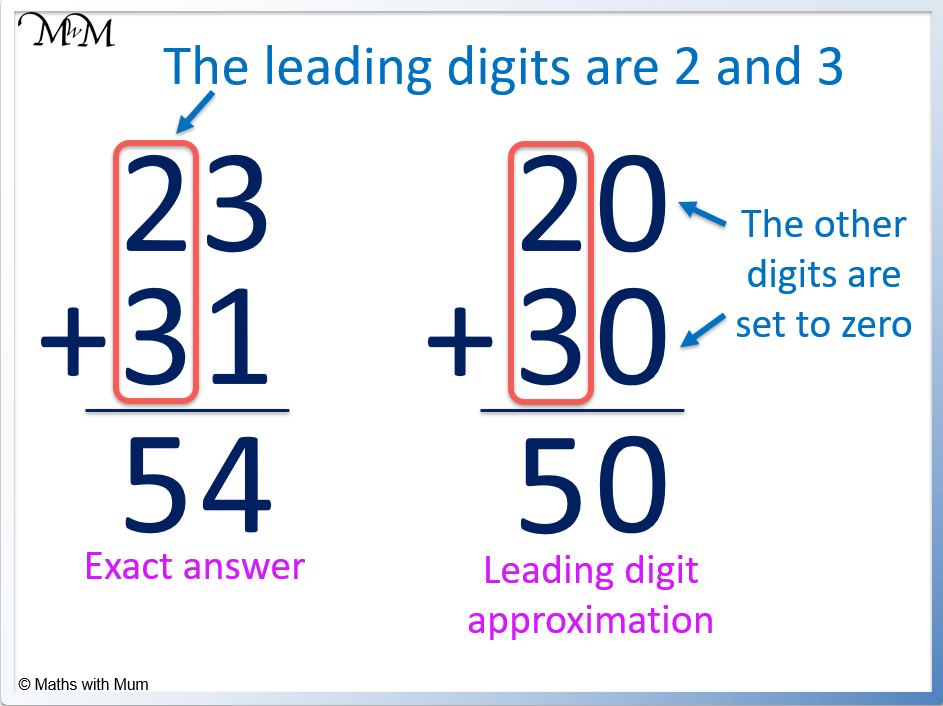• The leading digit is the first non-zero digit in a number.
• The leading digit in 23 is 2 and in 31 is 3.
• Leading digit approximation means to round the number to the leading digit and set the other digits to zero.
• 23 is approximated as 20 and 31 is approximated as 30.
• 23 + 31 = 54 which is the exact answer.
• 20 + 30 = 50 which is the leading digit approximation.

Look at the following digit to decide what the leading digit will round to.

If it is 5 or more, round up. If it is 4 or less, round down.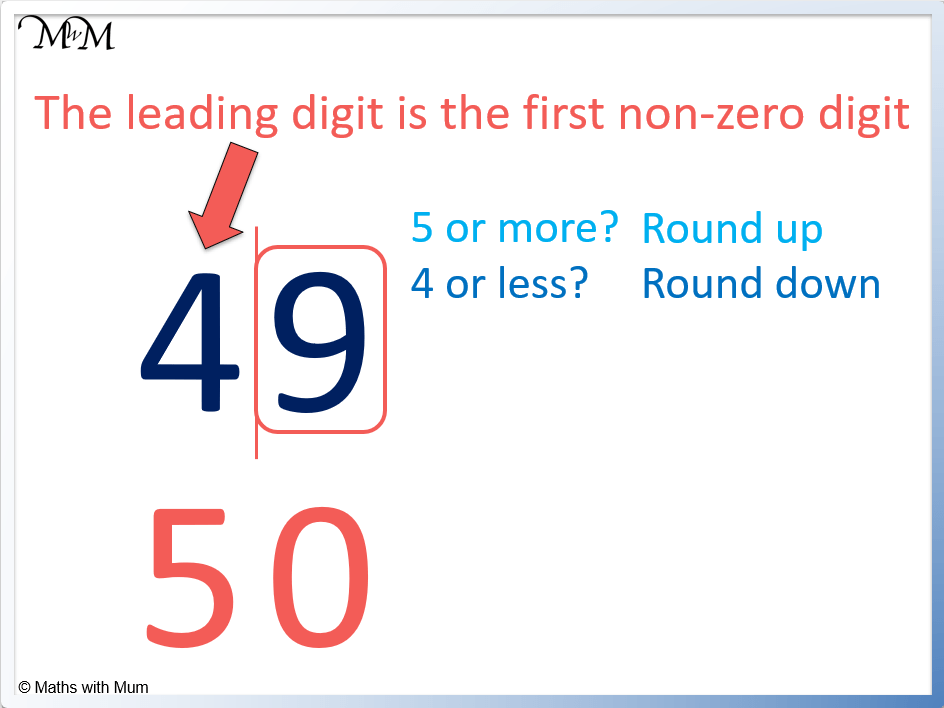• The leading digit of 49 is 4.
• We look at the digit after the 4 to decide if we round up or down.
• We look at the 9.
• If it is 5 or more, round up.
• If it is 4 or less, round down.
• 9 is ‘5 or more’ and so we round up.
• We round 49 up to 50.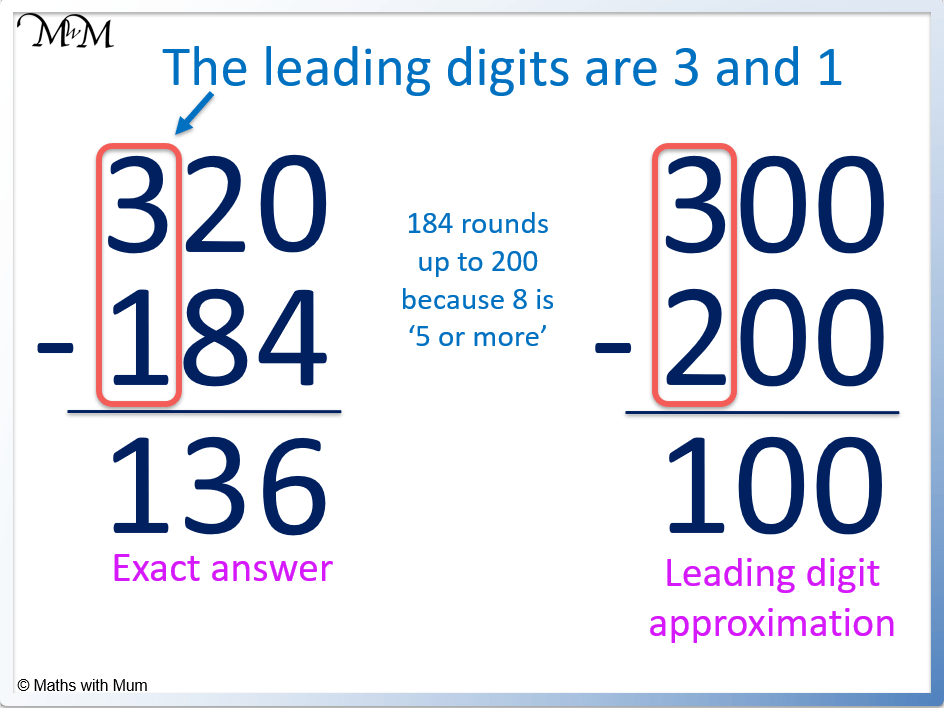• 320 has a leading digit of 3
• The next digit is 2, which is ‘4 or less’ so we round 320 down to 300.
• 184 has a leading digit of 1.
• The next digit is 8, which is ‘5 or more’ so we round 184 up to 200.
• The exact answer to 320 – 184 is 136.
• The leading digit approximation to 320 – 184 is 300 – 200 = 100.Supporting Lessons

## What is a Leading Digit of a Number?

The leading digit of a number is the first non-zero digit. For most numbers, the leading digit is simply the first digit. For example, the leading digit of 473 is 4. However, for decimal numbers starting with 0, the leading digit is the first digit that is not zero. For example, the leading digit of 0.0352 is the 3.

The leading digit of 473 is 4.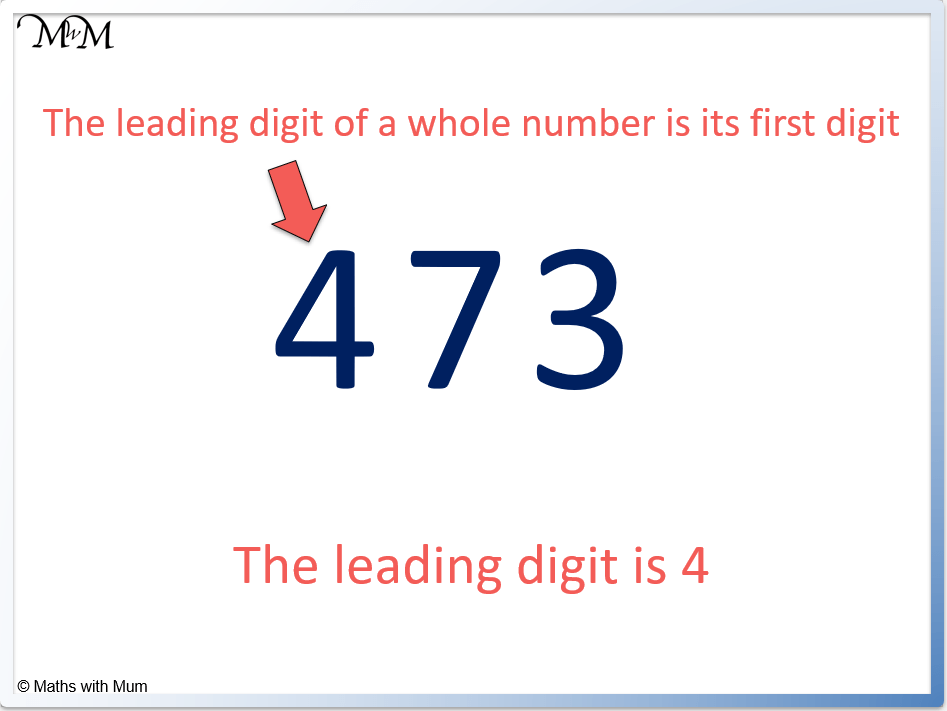For example, the leading digit of 23 is 2.#### The Leading Digit of a Decimal

The leading digit of a decimal number is the first digit in the number that is not a zero.

The leading digit of 0.0352 is 3.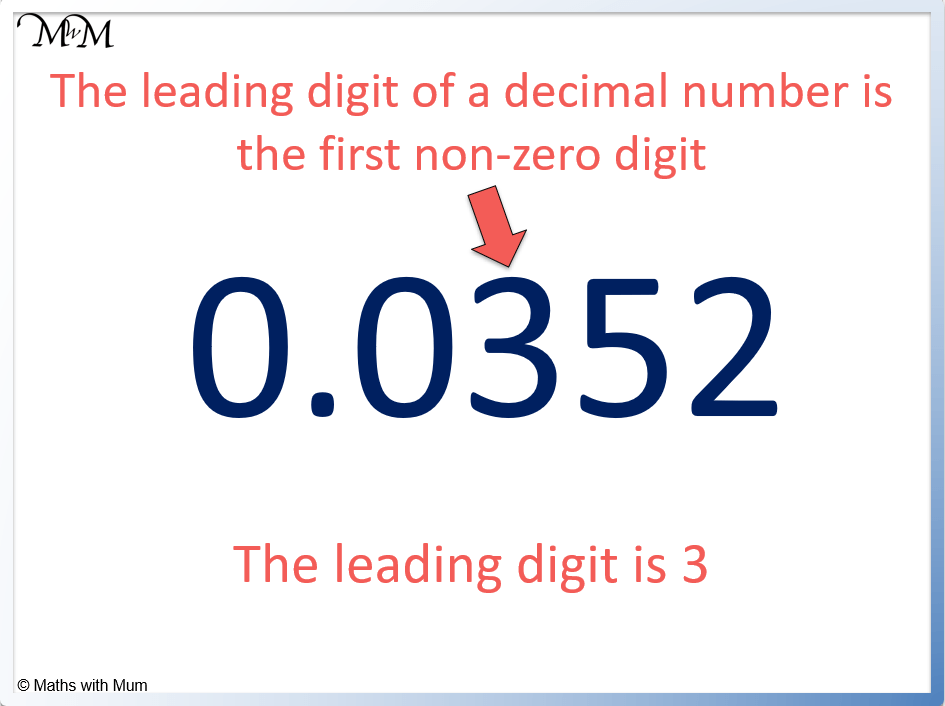For example, the leading digit of 0.000104 is 1.For example, the leading digit of 34.002 is 3. It is simply the first digit because the first digit is not a zero.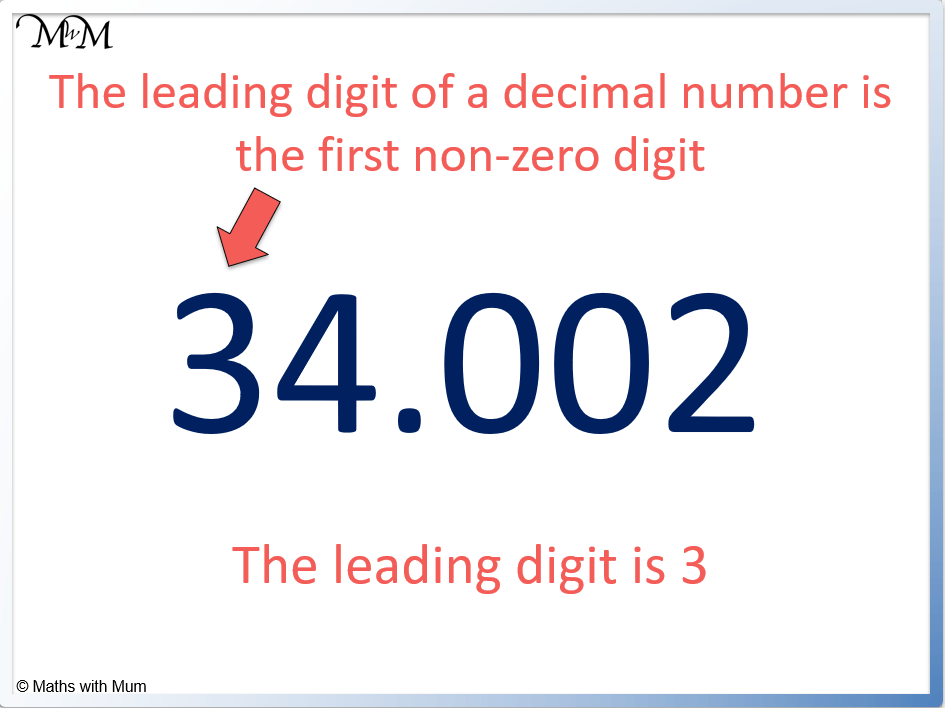## How to do Leading Digit Approximation

To round a number to its leading digit, first find the leading digit which is the first non-zero digit. Then look at the digit that comes immediately after it. If it is 5 or more, round the leading digit up to the next number. If it is 4 or less, leave the leading digit alone. Change all other digits to 0.

For example, find the leading digit approximation for 57. The choice is to round down to 50 or round up to 60.

The leading digit is the 5, so we look at the 7 to decide what to do.

7 is ‘5 or more’ so we round up to 60.For example, use leading digit estimation to round 148. The choice is round down to 100 or up to 200.

The leading digit is 1, so we look at the 4 to decide what to do.

4 is ‘4 or less’ so we round down. The leading digit stays as a 1 and the other digits become 0.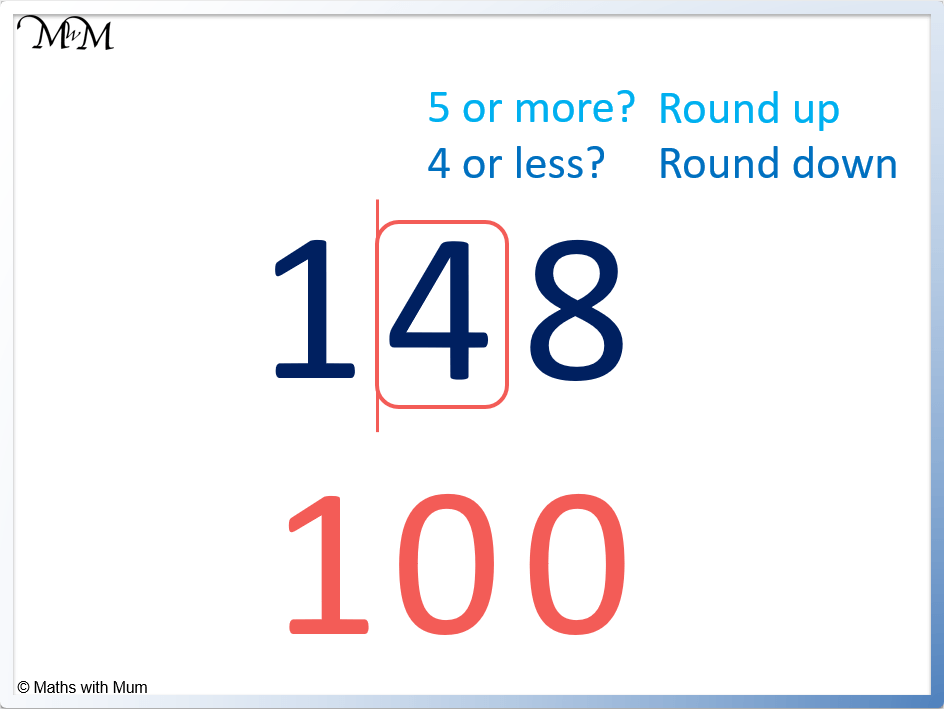Here is an example of using leading digit estimation on a decimal number 0.0797.

The leading digit is the 7 so we look at the 9 to decide whether to round up or down.

9 is ‘5 or more’ so we round up. The 7 becomes an 8 and we can set all other digits to 0.

0.0797 rounds up to 0.0800.

With decimal numbers, there is no need to write the 0 digits at the end of the number, so the number is written as 0.08.Leading digit approximation is used to estimate the answers to addition in order to make the addition easier. For example, 23 + 31 can be approximated as 20 + 30 which equals 50. The exact answer is 54.The addition is made easier using leading digit estimation because only the first digits need to be added rather than adding every digit in the number. The more digits an addition has, the easier it is to use leading digit approximation rather than adding the number fully.

## Leading Digit Approximation for Subtraction

Leading digit estimation can also be used to estimate a subtraction. For example 320 – 184 can be estimated as 300 – 200 which equals 100. The exact answer is 136.Now try our lesson on Rounding Decimals to the Nearest Whole Number where we learn how to round to the nearest whole number.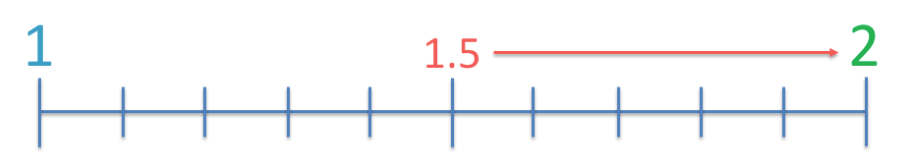error: Content is protected !!